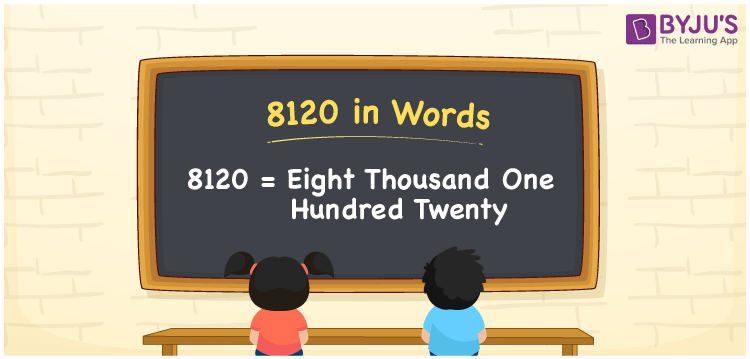# 8120 in Words

8120 in words is written as Eight thousand one hundred twenty. In both the International System of Numerals and the Indian System of Numerals, 8120 is written as Eight thousand one hundred twenty.

We often use 8120 as “8120 copies of this book have been sold”. 8120 represents a quantity, “how much of something” or “how many of something”. Hence, the number 8120 is a Cardinal Number.

 8120 in Words Eight thousand one hundred twenty Eight thousand one hundred twenty in Number 8120

## 8120 in English Words

8120 in English words is read as “Eight thousand one hundred twenty.”## How to Write 8120 in Words?

To write 8120 in words, we shall use the place value chart. In the place value chart, put 8 in the thousands, 1 in the hundreds, 2 in the tens and 0 in the ones, respectively. Let us make a place value chart to write the number 8120 in words.

 Thousands Hundreds Tens Ones 8 1 2 0

Thus, we can write the expanded form as

8 × Thousand + 1 × Hundred + 2 × Ten + 0 × One

= 8 × 8000 + 1 × 800 + 2 × 80 + 0 × 8

= 8000 + 100 + 20 + 0

= 8120

= Eight thousand one hundred twenty.

8120 is a natural number between 8119 and 8121.

8120 in words – Eight thousand one hundred twenty

• Is 8120 an odd number? – No
• Is 8120 an even number? – Yes
• Is 8120 a perfect square number? – No
• Is 8120 a perfect cube number? – No
• Is 8120 a prime number? – No
• Is 8120 a composite number? – Yes

## Frequently Asked Questions on 8120 in Words

Q1

### How to write 8120 in words?

8120 in words is written as Eight thousand one hundred twenty.
Q2

### How to write 8120 in words in the International and Indian System of Numerals?

In both, the system of numerals, 8120 in words, is written as Eight thousand one hundred twenty.
Q3

### How to write 8120 in a place value chart?

In the place value chart, write 8 in the thousands, 1 in the hundreds, 2 in the tens and 0 in the ones, respectively.